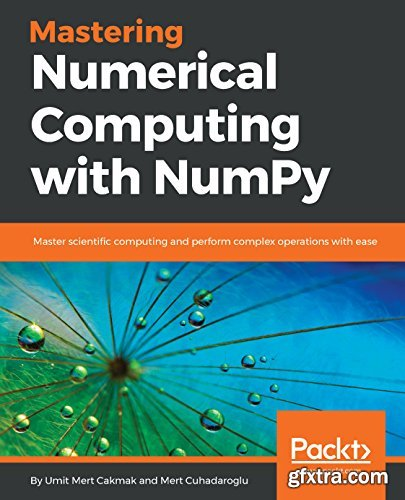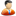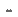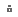﻿ Mastering Numerical Computing with NumPy » GFxtraMastering Numerical Computing with NumPy: Master scientific computing and perform complex operations with ease by Umit Mert Cakmak
English | 28 Jun. 2018 | ISBN: 1788993357 | 248 Pages | EPUB | 7.54 MB

Enhance the power of NumPy and start boosting your scientific computing capabilities

Key Features
Grasp all aspects of numerical computing and understand NumPy
Explore examples to learn exploratory data analysis (EDA), regression, and clustering
Access NumPy libraries and use performance benchmarking to select the right tool

Book Description
NumPy is one of the most important scientific computing libraries available for Python. Mastering Numerical Computing with NumPy teaches you how to achieve expert level competency to perform complex operations, with in-depth coverage of advanced concepts.

Beginning with NumPy's arrays and functions, you will familiarize yourself with linear algebra concepts to perform vector and matrix math operations. You will thoroughly understand and practice data processing, exploratory data analysis (EDA), and predictive modeling. You will then move on to working on practical examples which will teach you how to use NumPy statistics in order to explore US housing data and develop a predictive model using simple and multiple linear regression techniques. Once you have got to grips with the basics, you will explore unsupervised learning and clustering algorithms, followed by understanding how to write better NumPy code while keeping advanced considerations in mind. The book also demonstrates the use of different high-performance numerical computing libraries and their relationship with NumPy. You will study how to benchmark the performance of different configurations and choose the best for your system.

By the end of this book, you will have become an expert in handling and performing complex data manipulations.

What you will learn
Perform vector and matrix operations using NumPy
Perform exploratory data analysis (EDA) on US housing data
Develop a predictive model using simple and multiple linear regression
Understand unsupervised learning and clustering algorithms with practical use cases
Write better NumPy code and implement the algorithms from scratch
Perform benchmark tests to choose the best configuration for your system

Who This Book Is For
Mastering Numerical Computing with NumPy is for you if you are a Python programmer, data analyst, data engineer, or a data science enthusiast, who wants to master the intricacies of NumPy and build solutions for your numeric and scientific computational problems. You are expected to have familiarity with mathematics to get the most out of this book.

Working with NumPy Arrays
Linear Algebra with NumPy
Explanatory Data Analysis of US Housing Data withNumPy Statistics
Predicting Housing Prices Using Linear Regression
Clustering Clients of Wholesale Distributor Using NumPy
Python ML Squad: NumPy, SciPy, Pandas, Scikit-Learn
Overview of High-Performance Numerical Computing Libraries
Performance BenchmarksTO MAC USERS: If RAR password doesn't work, use this archive program:

RAR Expander 0.8.5 Beta 4  and extract password protected files without error.

TO WIN USERS: If RAR password doesn't work, use this archive program:

Latest Winrar  and extract password protected files without error.Solid   |

Information
Members of Guests cannot leave comments.